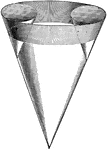### 3 Intersecting Cones

Illustration of the intersection of 3 cones.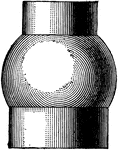### Intersecting Cylinder and Sphere

Illustration of the intersection of a cylinder and a sphere.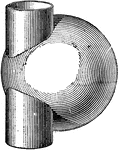### Intersecting Cylinder and Sphere

Illustration of the intersection of a cylinder and a sphere.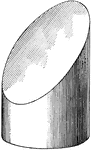### Plane Intersecting Cylinder

Illustration showing the intersection of a plane with a cylinder.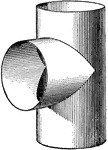### 2 Intersecting Cylinders

Illustration showing the intersection of two intersecting cylinders.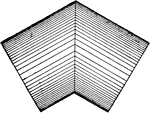### Intersecting Cylinders

Illustration of the intersection of 2 cylinders of equal diameter.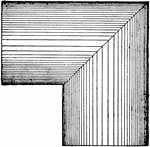### Intersecting Cylinders

Illustration of the intersection of 2 cylinders of equal diameter.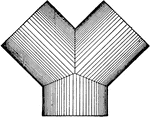### Intersecting Cylinders

Illustration of the intersection of 3 cylinders of equal diameter.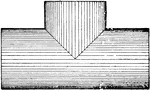### Intersecting Cylinders

Illustration of the intersection of 2 cylinders of equal diameter.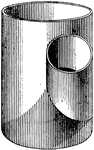### Intersecting Cylinders

Illustration of the intersection of 2 cylinders of unequal diameter.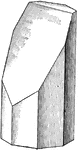### Plane Intersecting Octagonal Prism

Illustration showing the intersection of a plane with an octagonal prism.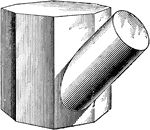### Intersecting Prism and Cylinder

Illustration of the intersection of an octagonal prism and a cylinder. The figures intersect at an oblique…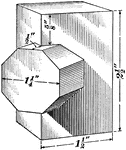### Intersecting Prisms

Illustration of the intersection of a quadrangular prism and an octagonal prism. The figures intersect…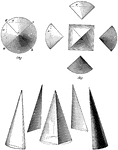### Relation Of Pyramid To Cone

Illustration showing the cone being treated as a many-sided pyramid.